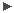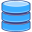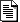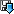# Common Trending and QC tools: Documentation

tqs = Trending and Quality Control Systemmake printable   new: see also:

v1.3: new: BasicPlot
v1.3.1: new: get_avgkeyval, get_avgfromhdrlist
v1.3.4: new: overexp, overexp_fromlist
v1.3.6: AssociationBlock: new method get_mcal
v1.3.8: AssociationBlock: new method get_prolist
v1.3.9: new: get_keyval
v1.3.10: improvements for ImagePlot and AssociationBlock
v1.3.11: new: get_arr
v1.3.14: new: get_wavearray
v1.4: new: BarPlot

- overview of pyQC
- pyQC script structuredatabases nonedfos tools processQC, writeQC, scoreQCoutput used by certifyProductsupload/download none

# pyQC: library qclib.py

## 0. Overview of content

 1. input/output def set_logging set logging level and format of logging messages class basic_options replaced by basic_parser, kept for backward compatability class wrapper_options replaced by wrapper_parser, kept for backward compatibility def print_message replaced by logging.info, etc.; kept for backward compatibility class get_options replaced by basic_parser, kept for backward compatability def get_from_NGAS download a raw, header, or product file from NGAS def find_fits find a fits file that has specified header keys def find_mcal find a fits file (calibration product) with specified PRO.CATG class ConfigFile representation of ASCII configuration file (DFOS style) class AssociationBlock representation of AB contents def get_confname get name of config file for importing script-specific configuration 2. mathematics/statistics def histogram returns histogram of 2D image [WH] def residuals returns residuals in Gaussian fit [WH] def pval returns Gauss function values [WH] def arr_median returns median of 2D image def arr_stddev returns standard deviation of 2D imaging def arr_MAD returns Median Absolute Deviation [WH] def im_divide image division taking care of division by zero 3. convenience functions to access fits header keys and data def get_keyval returns value of header key def get_avgkeyval returns average of two header key values def get_avgfromhdrlist returns average and rms of a keyword value across a list of headers def get_wavearray returns an array with wavelengths calculated from CRVAL, CRPIX, and CDELT def get_arr returns data array (image) from HDU and replaces NAN values with default value def overexp returns number of over-exposed pixels from a single frame def overexp_fromlist returns maximum number of over-exposed pixels from a list of frames 4. QC plotting class BasicPlot plots 1D data class LinePlot plots cuts through 2D images class HistoPlot plots histograms class ImagePlot plots 2D images for all extensions class SpecPlot plots 1D spectra (default scaling different from BasicPlot) class BarPlot plots data with error bars

## Command line parsers

Two command line parser are pre-defined.
Definitions:

# basic parsers with options used by all scripts
basic_parser.add_argument('-a', dest='ab', metavar='AB', help='name of AB', required=True, default=None)
# parser used by wrapper scripts
wrapper_parser.add_argument('-i', '--ingest', dest='ingest', action='store_true', default=False, help='ingest QC1 parameters')

Usage:

>>> parser = argparse.ArgumentParser(parents=[basic_parser], description='Example plotting script')
>>> parser.add_argument('--version', action='version', version='%(prog)s ' + _version_)
>>> # parse command line options and arguments
>>> args = parser.parse_args()
>>> # print AB name as given on command line when calling the script
>>> print args.ab

## set_logging

Definition:

def set_logging(level='info', format='')

level can be one of 'debug', 'info', 'warning', or 'error'

Usage:

>>> import logging
>>> set_logging(level='warning')
>>> logging.warning('this is a warning message')
script_XYZ [WARNING] this is a warning message
>>> logging.info('this is an info message')

The last command does not produce output since level='warning'.

## get_from_NGAS

Definition:

def get_from_NGAS(file, dir='', type='raw')

Usage:

>>> get_from_NGAS('CR_PDRK_090324A_DIT45.fits', dir='/data32/crires/calib/2009-03-24', type='product')

## find_fits

Definition:

Usage:

Returns file name of a fits file that has specified header key values, e.g.:

>>> file_name = find_fits(['HIERARCH ESO PRO CATG'], ['MASTER_BIAS'], dir='/data24/vimos/calib/2009-03-24')

## find_mcal

Definition:

def find_mcal(pro_catg, dir='')

Usage:

Returns file name of a fits product file that has specified PRO CATG, e.g.:

>>> file_name = find_mcal('CALPRO_THAT_CATALOG', dir='/data32/crires/calib/gen')

## ConfigFile

Definition:

class ConfigFile

Usage:

Needed by AssociationBlock. Assumes configuration files with DFOS-style syntax: <TAG> <VALUE1> <VALUE2> ...

## AssociationBlock

Definition:

class AssociationBlock(ConfigFile)

AssociationBlock is linked to the super class ConfigFile.

Usage:

>>> AB = AssociationBlock('CRIRE.2008-07-10T01:28:58.488_tpl.ab')

The complete AB content is read into a Python dictionary. Access it via the attribute 'content' as:

>>> print AB.content["DATE"]
'2008-07-09'

New with v1.3.14: the AB content can also be accessed directly via attributes as:

>>> print AB.date
'2008-07-09'

The output is a single character string. Multiple entries per tag are accessable via two nested Python lists:

>>> print AB.rawfile
[['/diska/data32/crires/raw/2008-07-09/CRIRE.2008-07-10T01:28:58.488.fits', 'DARK'], ['/diska/data32/crires/raw/2008-07-09/CRIRE.2008-07-10T01:30:09.615.fits', 'DARK']]

Environment variables appearing in the AB are expanded. Single elements from a list can be accessed as:

>>> print AB.rawfile
'/diska/data32/crires/raw/2008-07-09/CRIRE.2008-07-10T01:30:09.615.fits'

The class AssociationBlock offers methods for opening raw, header, and product files (by using the module PyFITS):

>>> rawHDUs = AB.get_raw()

This returns a list of header units (HDUs, each raw file is one HDU) that allow access to header keywords and data. Product files are opened in a similar way, the result is a dictionary with PRO_CATG as dictionary key:

>>> proHDUs = AB.get_pro()

With v1.1, this functions also with products (and product headers) in the dfo_calib and dfo_science directories [JP]. It is also possible to load raw file headers directly (as a Python dictionary with arcfile names as keys)

>>> rawHDRs = AB.get_hdr()

and to get raw files accessable via a dictionary (instead of a Python list):

>>> rawHDUs = AB.get_rawHDUs()

Examples:

54657.062611289999

>>> print proHDUs["CALPRO_DARK"].header["HIERARCH ESO QC DARKMED"] # QC parameter in first extension
0.666666666666667

## get_confname

Definition:

def get_confname(config_files, AB)

Usage:

Read the correct configuration file dependent on the RAW_TYPE in the AB:

>>> from config_cf import *
>>> module_name = get_confname(config_files, AB)
>>> exec 'from ' + module_name + ' import * '

## 2. Mathematics/statistics

Definitions:

def histogram(a, bins)
def residuals(p, y, x)
def pval(x, p)
def arr_median(arr)
def arr_stddev(arr, thres=5.0, median=None)
def im_divide(dividend, divisor)

These functions are short and in general self-explanatory.

## 3. Convenience functions for accessing fits header keys and data in fits files

Definitions:

def get_avgfromhdrlist(HDUlist, key1, key2='', default=-99, ext=0)
def get_arr(HDU, ext=0, default=0)
def overexp(HDU, ext=0, threshold=60000)

def overexp_fromlist(HDUlist, ext=0, threshold=60000)

These functions are short and in general self-explanatory.

## get_wavearray

Definition:

Usage:

Returns an array with wavelengths calculated from CRVAL<axis>, CRPIX<axis>, and CDELT<axis>. The lengths of the array is either NAXIS<axis> (if wshape <= 0) or equal to wshape. An optional offset can be added as a constant to all wavelengths. Example:

>>> spectrum = proHDUs['STD_EXTRACTED'].data # 1D image (=spectrum)
>>> pylab.plot(wavelengths, spectrum)

## BasicPlot

Definition:

class BasicPlot

Usage:

Plots 1D data, different datasets can be drawn in one plot with automated scaling. Examples:

>>> plot1 = BasicPlot() # initialize plot
>>> # add first dataset to plot, label it 'first set', plot colour is black
>>> # add a second dataset, plot in grey
>>> plot1.draw() # finally draw the plot

BasicPlot normally finds a reasonable scaling for x and y axes. If not, use:

>>> plot1.draw(xrange=[0,2048], yrange=[-10,10])

For defining the x values of the plot (default is 0, 1, 2, ...), pass an array with these values:

>>> xvals = numpy.arange(1024, 2048)
>>> plot1.draw(xarray=xvals)
.

## LinePlot

Definition:

class LinePlot(BasicPlot)

Usage:

Plots several column and/or row cuts of 2D images, allows averaging of columns and rows. Examples:

>>> plot1 = LinePlot() # initialize plot
>>> plot1.add_line(raw1_image, 'row', '@511', 'first raw', 0.00)
>>> # add row num. 512 to plot, label it 'first raw', plot colour is black (0.00)
>>> plot1.add_line(raw2_image, 'col', '@1023', 'last raw', 0.40)
>>> # add column 1024, plot in grey (0.40)
>>> plot1.add_line(mst_image, 'row', 'avg', 'avg rows', (0.00, 0.00, 1.00))
>>> # average of all rows, plot in blue
>>> plot1.draw() # finally draw the plot

LinePlot normally finds a reasonable scaling for x and y axes. If not, use:

>>> plot1.draw(xrange=[0,2048], yrange=[-10,10])

For defining the x values of the plot (default is 0, 1, 2, ...), pass an array with these values:

>>> xvals = numpy.arange(1024, 2048)
>>> plot1.draw(xarray=xvals)
.

## HistoPlot

Definition:

class histoPlot

Usage:

Plots a histogram of an image and adds a fit to the histogram.

>>> plot2 = histoPlot(logplot=True, stepsize=1, binrange=5)
>>> # logplot: plot logarithmic y axis (True/False)
>>> # stepsize: size of bins
>>> # binrange:
the histogram range (y axis) is given in multiples of the standard deviation of the image,
>>> # in this case: y axis = median - 5 * sigma, ..., median + 5 * sigma
>>> # plot the histogram of the image mst_hst, no fit (False)
>>> # plot the fit to the histogram (True), but not the
histogram itself
>>> plot2.draw()

## ImagePlot

Definition:

class imagePlot

Usage:

Plot several 2D images into one figure.

>>> mainplot = imagePlot(image_map=(4,1)) # initialize for 4x1 array
>>> mainplot.add_image(mstImgs, 1, 'MST DET 1') # first image, i.e. detector 1
>>> mainplot.add_image(mstImgs, 2, 'MST DET 2', cuts=[0.0, 10.0])
>>>
# define cuts explicitely
>>> mainplot.add_image(mstImgs, 3, 'MST DET 3', cuts=(3.0,))
>>> # define cuts as 3 sigma around median
>>> mainplot.add_image(mstImgs, 4, 'MST DET 4', cuts=(5.0,), xrange=(512,1024), yrange=(512,1024))
>>> # draw only a specific x and y area
>>> mainplot.draw() # finish
>>>
>>> mainplot = imagePlot(image_map=(1,2)) # initialize for 1x2 array
>>> # new with v1.1: compress image before plotting to save computing time
>>> # new with v1.2: define colour map
>>> mainplot.add_symbols([531, 608], [713, 599], 4)
>>> # new with v1.1: overplot symbols on last image
>>> mainplot.draw(aspect='equal') # new with v1.2: aspect='equal' or 'auto'

## SpecPlot

Definition:

class SpecPlot

Usage:

For plotting 1D data, functions with NumPy arrays.

>>> tbdata = proHDUs[catg_master].data
>>> # table data read from first extension of product file
>>> spec_opt = tbdata.field('Extracted_OPT')
>>> wavelength = tbdata.field('Wavelength')
>>> current_plot = SpecPlot()
>>> current_plot.add_spec(spec_rect, name='spectrum OPT', lin_col=(0.00, 0.00, 1.00)) # blue
>>> current_plot.draw(xarray=wavelength)

Scaling of axes is also supported (if default scaling is not sufficient):

>>> current_plot.draw(yrange=[0.0, 100.0])

## BarPlot

Definition:

class BarPlot

Usage:

For plotting multiple data sets with individual error bars. Example:

>>> wavelength = tbdata.field('wavelength')
>>> reso_ar = tbdata.field('resolution_ar')
>>> reso_rms_ar = tbdata.field('resolution_rms_ar')
>>> reso_ne = tbdata.field('resolution_ne')
>>> reso_rms_ne = tbdata.field('resolution_rms_ne')
>>> current_plot = BarPlot()
>>> current_plot.add_data(wavelength, reso_ar, reso_rms_ar, name='Ar lines', colour='b')
>>> current_plot.add_data(wavelength, reso_ne, reso_rms_ne, name='Ne lines', colour='r')
>>> current_plot.draw(xrange=[3500, 8000], yrange=[0, 10000])

>>> pylab.legend() # to make legend visible

A simpler example:

>>> current_plot = BarPlot()
>>> current_plot.add_data(x, y, yerr) # only one data set, default colour, no legend
>>> current_plot.draw() # default scaling usually gives good results

 Send comments to Last update: March 8, 2016